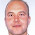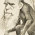# Learning Clojure

## Tuesday, January 25, 2011

### take-while-unstable

```;; I often find myself wanting to iterate something until it converges.

(defn collatz [n] (if (even? n) (recur (/ n 2)) (+ 1 (* 3 n))))

(iterate collatz 100) ; (100 76 58 88 34 52 40 16 4 4 4 4 4 4 ...)

;; And so I often find myself writing:

(defn take-while-unstable
([sq] (if (empty? sq) '()
(cons (first sq) (#'take-while-unstable (rest sq) (first sq)))))
([sq last] (cond (empty? sq) '()
(= (first sq) last) '()
:else (cons (first sq) (#'take-while-unstable (rest sq) (first sq))))))

;; Which allows me to stop the iteration once it's settled down.
(take-while-unstable (iterate collatz 100)) ; (100 76 58 88 34 52 40 16 4)

;; You can see how it works here:
(require 'clojure.contrib.trace)

(binding  [*print-length* 20] ; taking care not to look at the medusa
(clojure.contrib.trace/dotrace (take-while-unstable) (take-while-unstable (iterate collatz 100))))

;; TRACE t15884: (take-while-unstable (100 76 58 88 34 52 40 16 4 4 4 4 4 4 4 4 4 4 4 4 ...))
;; TRACE t15885: |    (take-while-unstable (76 58 88 34 52 40 16 4 4 4 4 4 4 4 4 4 4 4 4 4 ...) 100)
;; TRACE t15886: |    |    (take-while-unstable (58 88 34 52 40 16 4 4 4 4 4 4 4 4 4 4 4 4 4 4 ...) 76)
;; TRACE t15887: |    |    |    (take-while-unstable (88 34 52 40 16 4 4 4 4 4 4 4 4 4 4 4 4 4 4 4 ...) 58)
;; TRACE t15888: |    |    |    |    (take-while-unstable (34 52 40 16 4 4 4 4 4 4 4 4 4 4 4 4 4 4 4 4 ...) 88)
;; TRACE t15889: |    |    |    |    |    (take-while-unstable (52 40 16 4 4 4 4 4 4 4 4 4 4 4 4 4 4 4 4 4 ...) 34)
;; TRACE t15890: |    |    |    |    |    |    (take-while-unstable (40 16 4 4 4 4 4 4 4 4 4 4 4 4 4 4 4 4 4 4 ...) 52)
;; TRACE t15891: |    |    |    |    |    |    |    (take-while-unstable (16 4 4 4 4 4 4 4 4 4 4 4 4 4 4 4 4 4 4 4 ...) 40)
;; TRACE t15892: |    |    |    |    |    |    |    |    (take-while-unstable (4 4 4 4 4 4 4 4 4 4 4 4 4 4 4 4 4 4 4 4 ...) 16)
;; TRACE t15893: |    |    |    |    |    |    |    |    |    (take-while-unstable (4 4 4 4 4 4 4 4 4 4 4 4 4 4 4 4 4 4 4 4 ...) 4)
;; TRACE t15893: |    |    |    |    |    |    |    |    |    => ()
;; TRACE t15892: |    |    |    |    |    |    |    |    => (4)
;; TRACE t15891: |    |    |    |    |    |    |    => (16 4)
;; TRACE t15890: |    |    |    |    |    |    => (40 16 4)
;; TRACE t15889: |    |    |    |    |    => (52 40 16 4)
;; TRACE t15888: |    |    |    |    => (34 52 40 16 4)
;; TRACE t15887: |    |    |    => (88 34 52 40 16 4)
;; TRACE t15886: |    |    => (58 88 34 52 40 16 4)
;; TRACE t15885: |    => (76 58 88 34 52 40 16 4)
;; TRACE t15884: => (100 76 58 88 34 52 40 16 4)
;; (100 76 58 88 34 52 40 16 4)

;; Anyway, this function comes in so handy that I thought I'd lazyize it and give it some tests

(use 'clojure.test)

(with-test

(defn take-while-unstable
([sq] (lazy-seq (if-let [sq (seq sq)]
(cons (first sq) (take-while-unstable (rest sq) (first sq))))))
([sq last] (lazy-seq (if-let [sq (seq sq)]
(if (= (first sq) last) '() (take-while-unstable sq))))))

(is (= (take-while-unstable '()) '()))
(is (= (take-while-unstable '(1)) '(1)))
(is (= (take-while-unstable '(1 1)) '(1)))
(is (= (take-while-unstable '(1 2)) '(1 2)))
(is (= (take-while-unstable '(1 1 2)) '(1)))
(is (= (take-while-unstable '(1 1 1)) '(1)))
(is (= (take-while-unstable '(1 1 2 1)) '(1)))
(is (= (take-while-unstable '(1 2 3 4 5 6 7 7 7 7)) '(1 2 3 4 5 6 7)))
(is (= (take 10000 (take-while-unstable (range))) (take 10000 (range))))
(is (= (take-while-unstable (concat (take 10000 (range)) '(10000) (drop 10000 (range)))) (range 10001)))
(is (= (take-while-unstable '(nil)) '(nil)))
(is (= (take-while-unstable '(nil nil)) '(nil)))
(is (= (take-while-unstable '[nil nil]) '(nil)))
(is (= (take-while-unstable [ :a :b :c :d :d :e]) '(:a :b :c :d))))

(run-tests) ; {:type :summary, :test 1, :pass 14, :fail 0, :error 0}

;; Hopefully someone will find it useful. Can anyone break it?```

1.Mathematica has this same function, except it's called FixedPoint (and it has a cousin called FixedPointList). Mathematica actually has a kick ass library of higher order functions, so far the best I've seen of any functional language, though I'm by no means a guru.

But with Collatz you have cycles. A nifty higher order function would be one that efficiently detects cycles and terminates as soon as one is recognized. Would that have to be O(n)? I'm not sure, certainly one can always trade-off time for space by keeping pointers to previous iterates, like a rainbow table does.

Do you happen to know of any resources that specialize in enumerating and discussing some of these higher order functions? I feel like that is the next step to being a productive functional language programmer: having memorized tens or hundreds of these higher order functions as well as knowing exactly when and where they can be applied to simplify a problem.

2.It's probably got to be order infinity. I mean, has this sequence started cycling yet? 4 2 1 4 2 1 4 2 1 . . . .

If you're just looking for repeats, then (order (* range (log range))) sounds about right. An interesting question for Collatz.

Although of course for Collatz itself, all you need to check is whether the thing has come back to below its starting point.

Joy of Clojure's jolly good!
For maths look to the haskellloons.

3.4 2 1 4 2 1 ... has period 3, obviously, assuming x_n = f(x_{n-1}). So I suppose you were talking about systems where there is some hidden state that could cause the sequence to go haywire later on down the line. I'm just imagining iterating pure, deterministic functions.

The computational complexity I'm thinking of is: "if there is a repeat of length n after m steps, how quickly can you detect it?"

Advancing two pointers, one twice as fast as the other, and comparing if their values are ever equal, is an O(n) repeat-detector, with constant space complexity. But the constant in front of n is "2". I wonder if there is a better balance between space and time complexity if you have prior information about m and n.

4.Hmm, if we're talking about sequences like (iterate f x0) then that double-advance technique is very cool.

Surely it's O(n) space if you're keeping pointers, because you need to hold the sequence in between? But if the function's not expensive you could keep two separate values and iterate them separately, I think.

If the function is expensive then the best scheme may be to keep all the values so far in a b-tree or hash and look up each one to see if we've already been there.

Either way there'll be lots of operations per logical step, so I think the factor of 2 is a little optimistic.